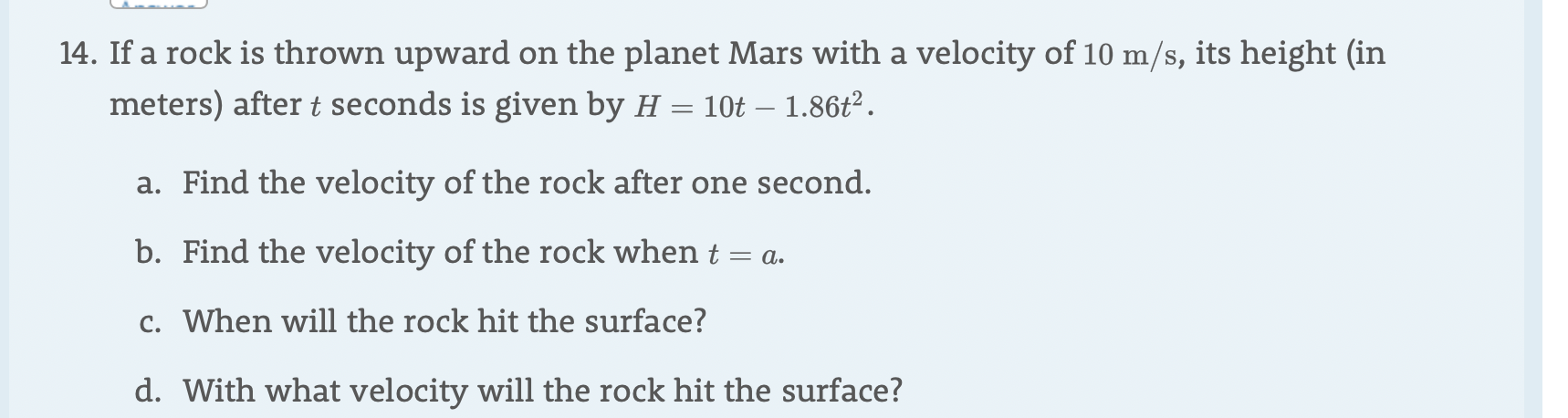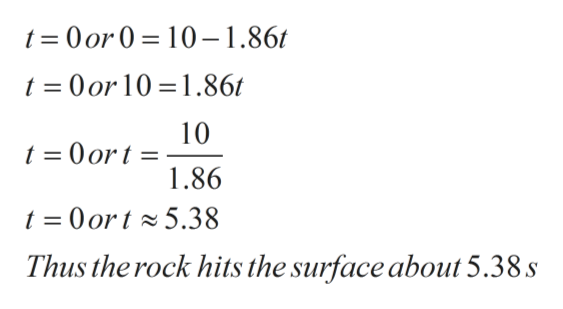14. If a rock is thrown upwardon the planet Mars with a velocity of 10 m/s, its height (inmeters) after t seconds is given by H = 10t - 1.86t2a. Find the velocity of the rock after one second.b. Find the velocity of the rock when t = a.c. When will the rock hit the surface?d. With what velocity will the rock hit the surface?

Questionhelp_outlineImage Transcriptionclose14. If a rock is thrown upward on the planet Mars with a velocity of 10 m/s, its height (in meters) after t seconds is given by H = 10t - 1.86t2 a. Find the velocity of the rock after one second. b. Find the velocity of the rock when t = a. c. When will the rock hit the surface? d. With what velocity will the rock hit the surface? fullscreen
Step 1

A rock is thrown  upward with a velocity of  10 m/s. Its  height ( in  meters)  after t seconds  is given by H= 10t-1.86t2..

c.  When  will be the rock  hit the surface?

d. With what  velocity with the  rock  hit the surface?

Step 2

Given H= 10t -1.86t2.

Rock will hit the ground  when H=0

Step 3

Plugging H=0 in above  equation and solving for t

0 = 10t-1.86t2

0 = t(10-1.86t)

Using &...help_outlineImage Transcriptioncloset 0or0 10-1.86t t 0 or 10 1.86t 10 =0ort = 1.86 t 0ort5.38 Thus the rock hits the surface about 5.38.s fullscreen

Want to see the full answer?

See Solution

Want to see this answer and more?

Our solutions are written by experts, many with advanced degrees, and available 24/7

See Solution
Tagged in

Calculus